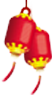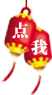# 经典Java高级算法笔试题，含代码

题目：古典问题：有一对兔子，从出生后第3个月起每个月都生一对兔子，小兔子长到第三个月后每个月又生一对兔子，假如兔子都不死，问每个月的兔子总数为多少？

//这是一个菲波拉契数列问题

```　　publicclasstest01{

publicstaticvoidmain(String[]args){

intf1=1,f2=1,f;

intM=30;

System.out.println(1);

System.out.println(2);

for(inti=3;i<M;i++){

f=f2;

f2=f1+f2;

f1=f;

System.out.println(f2);

}

}

}```

题目：判断101-200之间有多少个素数，并输出所有素数。

程序分析：判断素数的方法：用一个数分别去除2到sqrt(这个数)，如果能被整除，则表明此数不是素数，反之是素数。

```　　publicclasstest02{

publicstaticvoidmain(String[]args){

intcount=0;

for(inti=101;i<200;i+=2){

booleanflag=true;

for(intj=2;j<=Math.sqrt(i);j++){

if(i%j==0){

flag=false;

break;

}

}

if(flag==true){

count++;

System.out.println(i);

}

}

System.out.println(count);

}

}```

题目：求s=a+aa+aaa+aaaa+aa…a的值，其中a是一个数字。例如2+22+222+2222+22222(此时共有5个数相加)，几个数相加有键盘控制。

```　　importjava.util.Scanner;

publicclasstest08{

publicstaticvoidmain(String[]args){

Scannerinput=newScanner(System.in);

inta=input.nextInt();

intn=input.nextInt();

intsum=0,b=0;

for(inti=0;i<n;i++){

b+=a;

sum+=b;

a=a*10;

}

System.out.println(sum);

}

}```

题目：一个数如果恰好等于它的因子之和，这个数就称为"完数"。例如6=1＋2＋3.编程找出1000以内的所有完数。

```　　publicclasstest09{

publicstaticvoidmain(String[]args){

for(inti=1;i<=1000;i++){

intt=0;

for(intj=1;j<=i/2;j++){

if(i%j==0){

t+=j;

}

}

if(t==i){

System.out.println(i);

}

}

}

}```

题目：一球从100米高度自由落下，每次落地后反跳回原高度的一半；再落下，求它在第10次落地时，共经过多少米？第10次反弹多高？

```　　publicclasstest10{

publicstaticvoidmain(String[]args){

doubleh=100;

doubles=100;

for(inti=1;i<=10;i++){

h=h/2;

s=s+2*h;

}

System.out.println(s);

System.out.println(h);

}

}```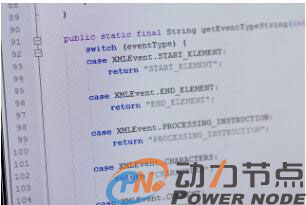以上就是动力节点java培训机构的小编针对“经典Java高级算法笔试题，含代码”的内容进行的回答，希望对大家有所帮助，如有疑问，请在线咨询，有专业老师随时为你服务。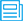相关文章推荐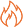HOT资讯 >

• 姓名
• 手机
• 性别
• 意向课程